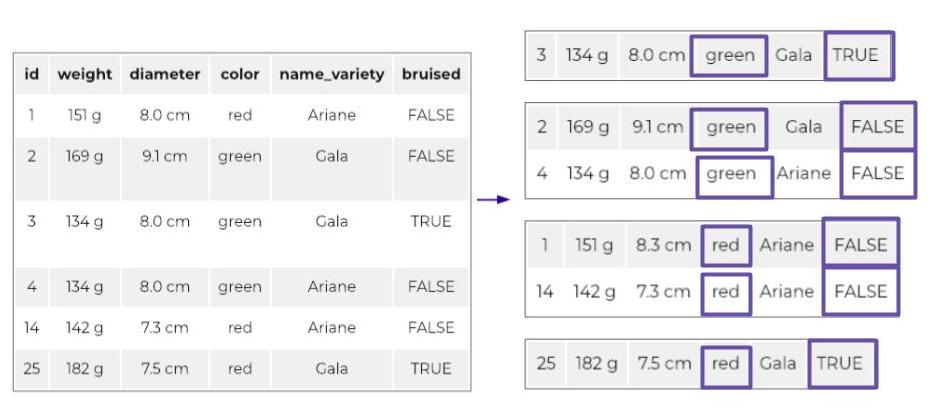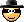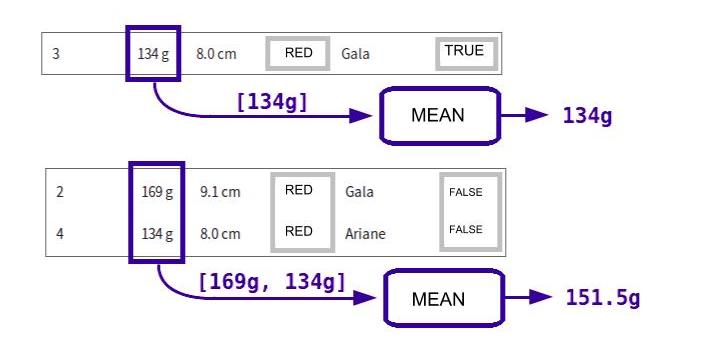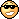Last updated on 4/19/21

## Aggregate rows in a table

Log in or subscribe for free to enjoy all this course has to offer!Aggregation is used to perform computations over multiple rows of a table. When we do this, we say that we are aggregating these rows—that is, forming aggregates in order to perform an operation on them.

For example, suppose I ask the following question ...

What is the average weight of each color of apple?

I need to calculate a value for each aggregate:

• one value (the average weight) for multiple apples (all of the green apples)

• one value (the average weight) for all of the red apples

• etc.

As you have guessed, we will calculate as many average weight values as there are colors of apples.

Are you beginning to see the importance of aggregation?

A proper aggregation consists of two steps, and therefore has two elements:

1. a group of partitioning attributes (such as color)

2. one (or more) aggregate function(s) (such as average)

#### Partition

If I partition my apples by color, this means I form groups of apples (called aggregates), and the apples within each group all have the same color.

I can also partition by multiple attributes. For example, let’s say that I add an attribute to my apple table called bruised, a Boolean attribute that is  ` TRUE`  if the apple is bruised. I can partition my apples on the basis of both attributes, color and bruised. The result will be groups within which all of the apples have the same color and the same value for the bruised attribute:Partition by [color, bruised]

#### Apply aggregate functions

Once the aggregates are formed, you need to do something with them!

This is where aggregate functions come in.Their role is to take a group of multiple rows, perform a calculation on them, and return a single value for each group.

For example, you could apply a function to calculate the average weight of each group of apples—first the group of bruised green apples, then the group of non-bruised yellow apples, etc.Generally, the aggregate function takes a list and returns a single value. For example, to calculate the  average  price per kilo of the apples, the  ` average`  function would take the weights of the apples in the aggregate, and return the average value for this list. That’s what’s happening in the image above.

#### The result

Here is the result of an aggregation operation with partitioning by the attributes color and bruised, in which two aggregate functions are applied.

1. the  `average`  function (abbreviated avg), applied to the weight of the apples

2. the  `count`  function, which counts the number of apples in the aggregate

 color bruised avg (weight) count green True 134 g 1 green False 151.5 g 2 red False 146.5 g 2 red True 182 g 1

According to this result, the average weight of the two green, non-bruised apples is 151.5 grams. Cool, no?We’ll be looking ahead to the day when you are confronted with a Big Data problem, involving a huge data set that needs to be processed.

When the data set is too large, several computers (servers) are often used to process the data in parallel—it goes much faster! You need only one server to coordinate the others and distribute the data judiciously among the parallel servers. One way to approach this is to use the MapReduce technique.

With MapReduce, the information is first divided into small chunks. Each chunk has a key and a value. Next, a reduce function is defined. When it’s time to execute the calculation, all of the chunks of information that have a particular key are directed to the same parallel server. This server then applies the reduce function to all of the values of the chunks it receives that have the same key.

MapReduce works in exactly the same way as aggregation. Here is how they correspond:

 Map Reduce Aggregation Information chunk 1 row of a table Key Partitioning attributes Value Attributes sent to the aggregate function Reduce Function Aggregate Function

Take another look at the above illustration, which explains partitioning by attributes. The right side of the graphic shows a table divided into several parts. MapReduce directs each of these parts to a different server. The server then applies the reduce function, which is, for example, the average function.

It’s as simple as that!

• The two parts to an aggregation are:

• partitioning attributes

• aggregate function(s)

• A basic aggregate function takes multiple input values and returns a single value.

• Aggregation results in a table with fewer rows (as many rows as aggregates)...

• ... and these rows do not represent the same objects as the original table, with the latter representing groups of rows based on a partitioning attribute.